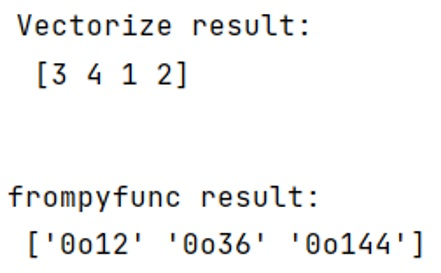# Difference between numpy.frompyfunc() and numpy.vectorize() functions

Learn about the difference between numpy.frompyfunc() and numpy.vectorize() functions in Python.
Submitted by Pranit Sharma, on March 02, 2023

NumPy is an abbreviated form of Numerical Python. It is used for different types of scientific operations in python. Numpy is a vast library in python which is used for almost every kind of scientific or mathematical operation. It is itself an array which is a collection of various methods and functions for processing the arrays.

## numpy.frompyfunc() Vs numpy.vectorize() functions

The numpy.frompyfunc() takes an arbitrary Python function and returns a NumPy ufunc (universal function). It can be used, for example, to add broadcasting to a built-in Python function.

On the other hand, numpy.vectorize() is a generalized function class. It defines a vectorized function that takes a nested sequence of objects or numpy arrays as inputs and returns a single numpy array or a tuple of numpy arrays. The vectorized() function evaluates pyfunc over successive tuples of the input arrays like the Python map function, except it uses the broadcasting rules of numpy.

The data type of the output vectorized is determined by calling the function with the first element of the input. This can be avoided by specifying the otypes of argument.

Let us understand with the help of an example,

## Python code to demonstrate the difference between numpy.frompyfunc() and numpy.vectorize() functions

```# Import numpy
import numpy as np

# Defining a function
def fun(a,b):
if a > b:
return a - b
else:
return a + b

# Using np.vectorize
res = np.vectorize(fun)

# Creating a sequence of objects
objs = [1,2,3,4]

# Display result
print("Vectorize result:\n",res(objs,2),"\n")

# Using frompyfunc
res = np.frompyfunc(oct, 1, 1)

# Display result
print("frompyfunc result:\n",res(np.array((10, 30, 100))))
```

Output:Languages: » C » C++ » C++ STL » Java » Data Structure » C#.Net » Android » Kotlin » SQL
Web Technologies: » PHP » Python » JavaScript » CSS » Ajax » Node.js » Web programming/HTML
Solved programs: » C » C++ » DS » Java » C#
Aptitude que. & ans.: » C » C++ » Java » DBMS
Interview que. & ans.: » C » Embedded C » Java » SEO » HR
CS Subjects: » CS Basics » O.S. » Networks » DBMS » Embedded Systems » Cloud Computing
» Machine learning » CS Organizations » Linux » DOS
More: » Articles » Puzzles » News/Updates